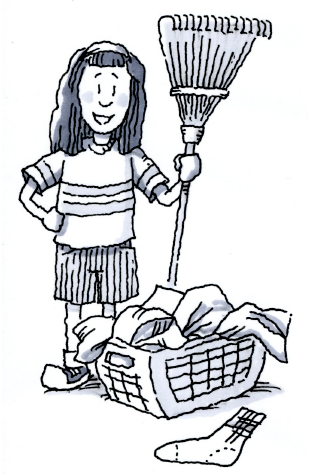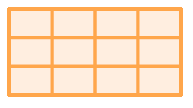Home > CC2MN > Chapter cc22 > Lesson cc22.2.3 > Problem2-66

2-66.On Saturday, Stella worked for two hours helping her mother around the house. She spent $\frac { 1 } { 3 }$ of her time doing laundry, $\frac { 1 } { 4 }$ of her time cleaning, and the rest of her time working in the yard. How much of her time was spent in the yard? Show all of your work. Use a diagram if it helps. Homework Help ✎

Draw a rectangle like the one the right.

How can you subdivide it so you can represent one third and one fourth?
Try this before moving on.

One way to show both fractions is with a diagram:

Copy this diagram and shade $\frac{1}{3}$ and $\frac{1}{4}$.

What is left?

The answer is $\frac{5}{12}$ or $50$ minutes.

How can you arrive at this answer without drawing a diagram?